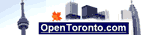# L/100km Calculator

Fuel spent liters (L) or US gallons:

Kilometers (km) or miles (mi) driven:

Select units type (metric or US imperial):

Rounding options:

 Fuel economy is the amount of fuel required to move a vehicle over a given distance. Fuel efficiency is the efficiency of converting energy contained in a carrier fuel to kinetic energy or work. Fuel efficiency can also mean the output one gets for a unit amount of fuel input such as "miles per gallon" or "liters per 100 kilometers" for an automobile (sometimes called fuel economy).  Automobile fuel efficiency can be calculated in either l/100 km or MPG  (Litres per 100 km).

Deutsch   English   Espanol   Francais   Italiano   Portuguese

Calculators:
MPG calculator
L/100km fuel calculator
L/100km to MPG calculator
MPG to L/100km conversion
Miles to km conversion
mph to kmh conversion
Gallon to Litre Conversion
Pounds to kilograms conversion
Celsius to Fahrenheit
Fahrenheit to Celsius conversion
Recommended Daily Calorie Calculator
BMI Calculator
Ounces to grams conversion
Yards to mm conversion
Yards to meters Conversion
Feet to meters Conversion
Inches to mm Conversion
Time in Hamilton, Ontario